# Dropped sheets

Three consecutive sheets dropped from the book. The sum of the numbers on the pages of the dropped sheets is 273. What number has the last page of the dropped sheets?

x =  48

### Step-by-step explanation:

x+(x-1)+(x-2)+(x-3)+(x-4)+(x-5)=273

6x = 288

x = 48

Our simple equation calculator calculates it.Did you find an error or inaccuracy? Feel free to write us. Thank you!Tips to related online calculators
Do you have a linear equation or system of equations and looking for its solution? Or do you have a quadratic equation?

## Related math problems and questions:

• Last pageTwo consecutive sheets dropped out of the book. The sum of the numbers on the sides of the dropped sheets is 154. What is the number of the last page of the dropped sheets?
• Claire and bookAfter Claire has read the first 5/8 of the book, there are 120 pages left to read. What is the total number of pages of the book?
• 1 pageOne page is torn from the book. The sum of the page numbers of all the remaining pages is 15,000. What numbers did the pages have on the page that was torn from the book?
• Book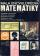To number pages of the thick book was used 4201 digits. How many pages has this book?
• A bookA book contains 524 pages. If it is known that a person will select any one page between the pages numbered 125 and 384, find the probability of choosing the page numbered 252 or 253.
• The sumThe sum of five consecutive odd numbers is 75. Find out the sum of the second and fourth of them.
• Janka and Danka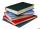Janka and Danka began to read the same day the books. Janka had 276 pages, Danka 204 pages. They agreed that both read every day the same number of pages until theirs books read. A) How many maximum pages they had to read the day to honor their agreement?
• John SmithJohn Smith is reading a 435-page novel. If he reads the same number of pages per day and the number is a prime number greater than 10, how many pages per day will he read?
• Every day 7 pagesAdelka reads the book 7 pages every day. When she reads one more page a day, she will read it three days earlier. How long will Adelka read a book? How much does a book of pages have?
• Borrowed book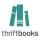Jane must as soon as possible return a borrowed book. She figured that when she read 15 pages a day return book in time. Then she read 18 pages a day and then return the book one day before. How many pages should have a book?
• Pages countingThere are pages numbered from 2 to 104 in the book. How many digits have to be printed to number the pages?
• Book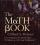John read the book so that it read 30 pages per day. Maxim read 34 pages a day and read it two days earlier than John. How many pages has the book?
• Sum of four numbers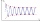The sum of four consecutive natural numbers is 114. Find them.
• Even / odd numbers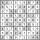a / Using variable n write two consecutive odd numbers. b / the sum of three consecutive odd numbers is 333. What are this numbers?
• Book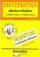Calculate the thickness of the page of the collection of examples from mathematics, where 4.87 cm thick and has 451 pages.
• Unknown number4/5 of a number is 276. what is 2/3 of the same number?
• Unknown numberIf I reduced the sum of the numbers 70 and the unknown number three times, I would get 100. what is the unknown number?